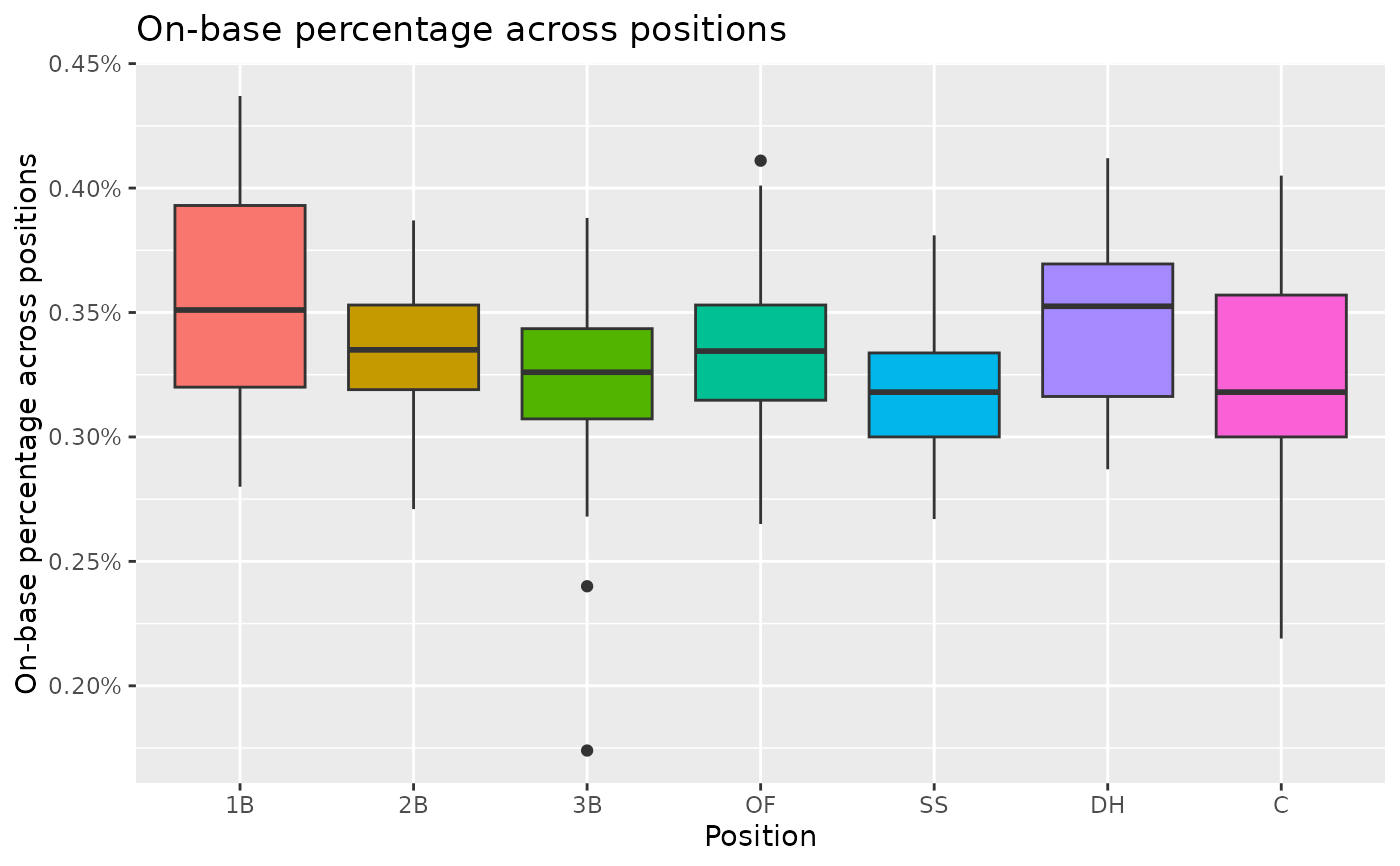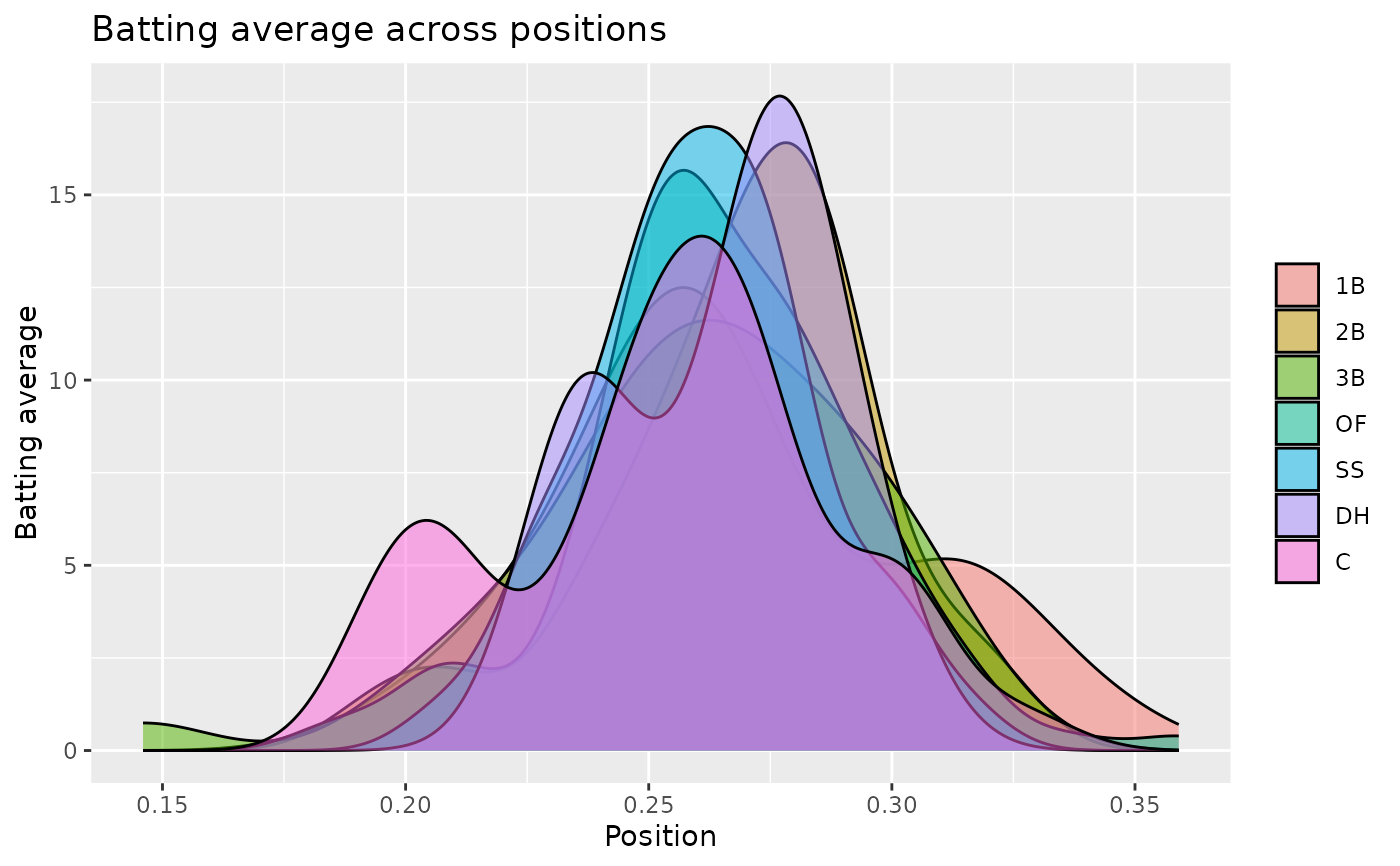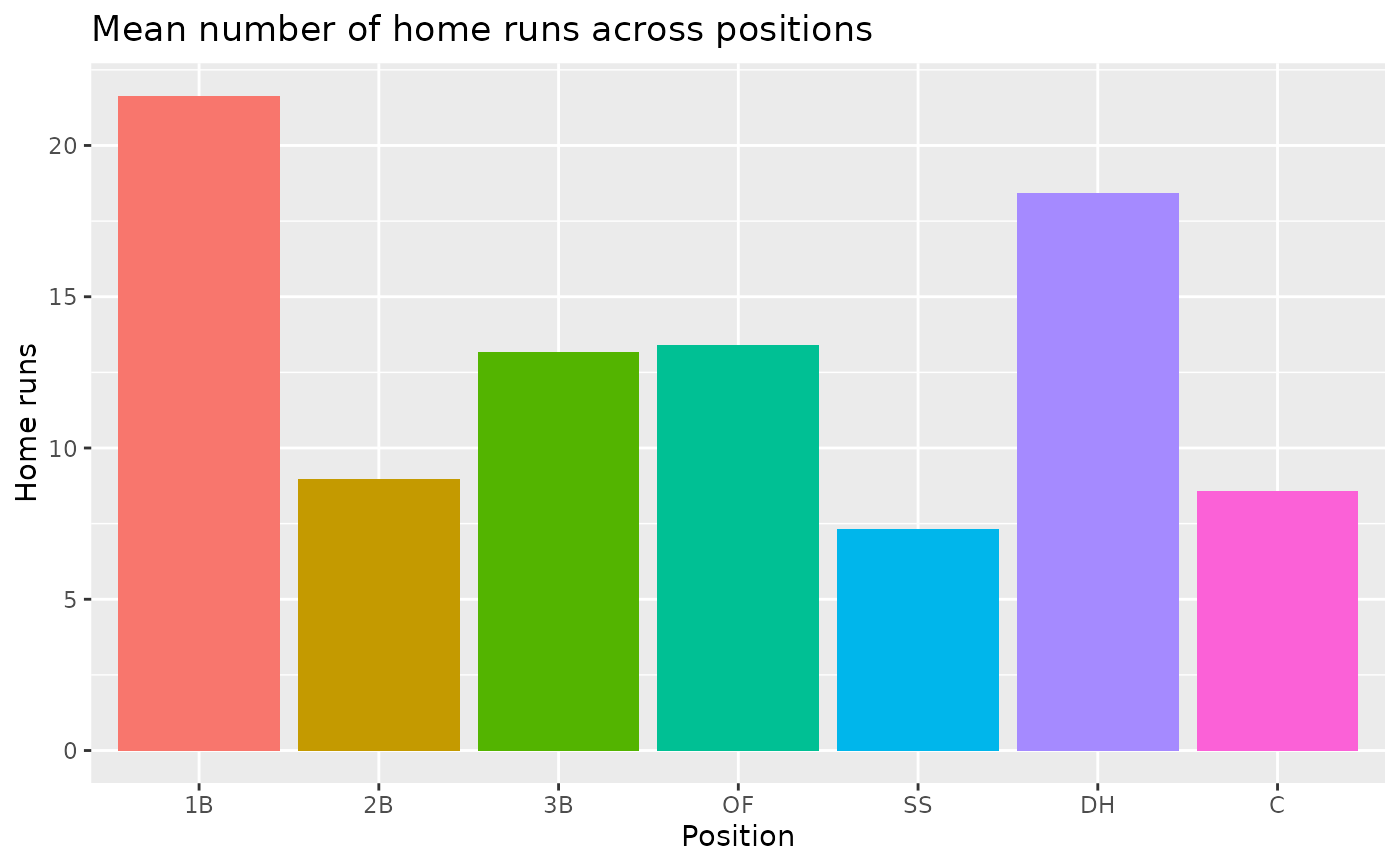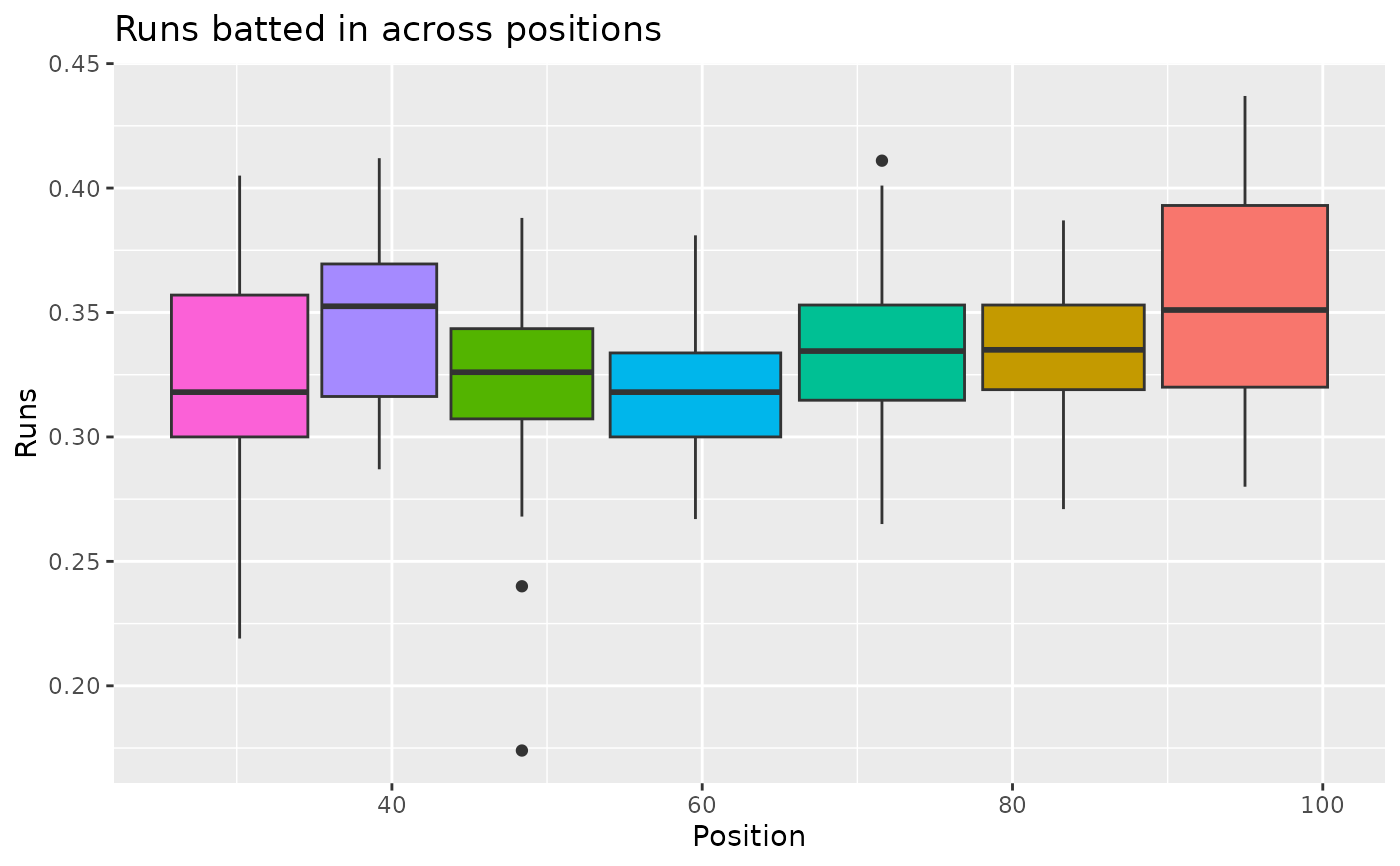Major League Baseball Player Hitting Statistics for 2010.

## Usage

mlbbat10

## Format

A data frame with 1199 observations on the following 19 variables.

name

Player name

team

Team abbreviation

position

Player position

game

Number of games

at_bat

Number of at bats

run

Number of runs

hit

Number of hits

double

Number of doubles

triple

Number of triples

home_run

Number of home runs

rbi

Number of runs batted in

total_base

Total bases, computed as 3HR + 23B + 1*2B + H

walk

Number of walks

strike_out

Number of strikeouts

stolen_base

Number of stolen bases

caught_stealing

Number of times caught stealing

obp

On base percentage

slg

Slugging percentage (total_base / at_bat)

bat_avg

Batting average

## Source

https://www.mlb.com, retrieved 2011-04-22.

## Examples


library(ggplot2)
library(dplyr)
library(scales)

mlbbat10_200 <- mlbbat10 %>%
filter(mlbbat10\$at_bat > 200)

# On-base percentage across positions
ggplot(mlbbat10_200, aes(x = position, y = obp, fill = position)) +
geom_boxplot(show.legend = FALSE) +
scale_y_continuous(labels = label_number(suffix = "%", accuracy = 0.01)) +
labs(
title = "On-base percentage across positions",
y = "On-base percentage across positions",
x = "Position"
)# Batting average across positions
ggplot(mlbbat10_200, aes(x = bat_avg, fill = position)) +
geom_density(alpha = 0.5) +
labs(
title = "Batting average across positions",
fill = NULL,
y = "Batting average",
x = "Position"
)# Mean number of home runs across positions
mlbbat10_200 %>%
group_by(position) %>%
summarise(mean_home_run = mean(home_run)) %>%
ggplot(aes(x = position, y = mean_home_run, fill = position)) +
geom_col(show.legend = FALSE) +
labs(
title = "Mean number of home runs across positions",
y = "Home runs",
x = "Position"
)# Runs batted in across positions
ggplot(mlbbat10_200, aes(x = run, y = obp, fill = position)) +
geom_boxplot(show.legend = FALSE) +
labs(
title = "Runs batted in across positions",
y = "Runs",
x = "Position"
)